# JavaSE基础——（16）List集合

三、栈和队列

5.1泛型的基本概述

5.2泛型的好处

5.3泛型的使用

5.4ArrayList存储字符串使用泛型遍历

5.5泛型的由来

6.1泛型类概述与使用

6.2泛型方法概述与使用

6.3泛型通配符

7.1增强for循环的概述和使用

7.2三种迭代是否能删除

9.1可变参数概述与使用

9.2Arrays工具类的asList()方法

import java.util.ArrayList;
import java.util.Iterator;
public class ListTest {
public static void main(String []args){
ArrayList list=new ArrayList();
list.add("a");
list.add("a");
list.add("b");
list.add("b");
list.add("b");
list.add("c");
list.add("c");
ArrayList newList=getSingle(list);
System.out.println(newList);
}
public static ArrayList getSingle(ArrayList list){
ArrayList newList=new ArrayList<>();
Iterator it=list.iterator();
while(it.hasNext()){
Object obj=it.next();
if(!newList.contains(obj)){
newList.add(obj);
}
}
return newList;
}
}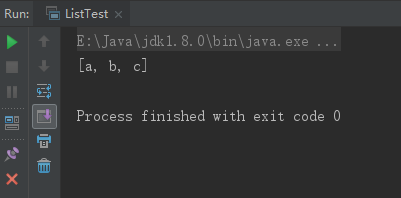public void addFirst(E e)//将元素添加到链表头部
public void addLast(E e)//将元素添加到链表尾部
public E getFirst()//获取链表头部元素
public E getLast()//获取链表尾部元素
public E removeFirst()//删除链表头部元素，并返回删除的元素
public E removeLast()//删除链表尾部元素，并返回删除的元素
public E get(int index)//获取指定索引处的元素

三、栈和队列

import java.util.LinkedList;
public class ListTest {
public static void main(String []args){
Stack stack=new Stack();
stack.in("a");
stack.in("b");
stack.in("c");
while(!stack.isEmpty()){
System.out.println(stack.out());
}
}
}
class Stack{
private LinkedList list=new LinkedList();
//模拟进栈
public void in(Object obj){
list.add(obj);
}
//模拟出栈
public Object out(){
return list.removeLast();
}
//判断栈元素是否为空
public boolean isEmpty(){
return list.isEmpty();
}
}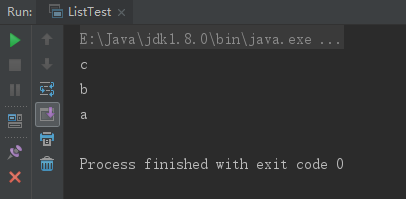5.1泛型的基本概述

5.2泛型的好处

5.3泛型的使用
public class PatternTest {
public static void main(String []args){
ArrayList<Student> list=new ArrayList<Student>();
list.add(new Student("测试1",20));
list.add(new Student("测试2",21));
list.add(new Student("测试3",22));
list.add(new Student("测试4",23));
Iterator<Student> it=list.iterator();
while(it.hasNext()){
Student stu=it.next();
System.out.println(stu.getName()+" "+stu.getAge());
}
}
}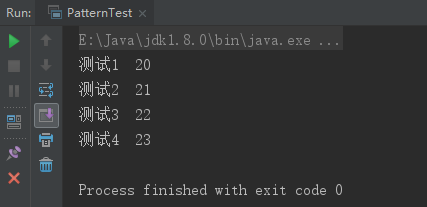5.4ArrayList存储字符串使用泛型遍历
public class PatternTest {
public static void main(String []args){
ArrayList<String> list=new ArrayList<>();
list.add("a");
list.add("b");
list.add("c");
Iterator<String> it=list.iterator();
while(it.hasNext()){
System.out.println(it.next());
}
}
}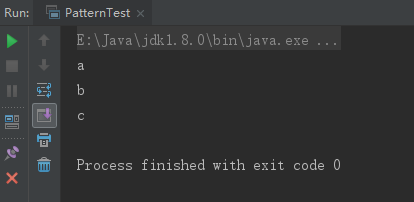5.5泛型的由来

6.1泛型类概述与使用

class myPattern<T>{
private T t;
public myPattern(T t) {
this.t=t;
}
public T getT() {
return t;
}
public void setT(T t) {
this.t = t;
}
}

6.2泛型方法概述与使用

public <泛型类型> 返回值类型 方法名(泛型类型 形参名)

public <T> void show(T t){
System.out.println(t);
}

6.3泛型通配符

List<?> list=new ArratList<String>();//当右边的泛型是不确定时，左边可以指定为？

7.1增强for循环的概述和使用

//遍历数组
int arr[]={1,2,3};
for(int i:arr){
System.out.println(i);
}
//遍历集合
ArrayList<String> list=new ArrayList<String>();
list.add("a");
list.add("b");
list.add("c");
for(String i:list){
System.out.println(i);
}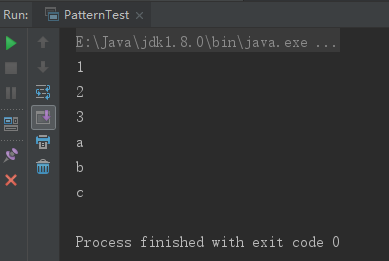7.2三种迭代是否能删除

ArrayList<String> list=new ArrayList<String>();
list.add("a");
list.add("b");
list.add("b");
list.add("c");
//for循环删除，索引要--
for(int i=0;i<list.size();i++){
if("b".equals(list.get(i))){
list.remove(i--);
}
}
//迭代器删除，用迭代器自身的remove方法
Iterator<String> it=list.iterator();
while(it.hasNext()){
if("b".equals(it.next())){
it.remove();
}
}
//增强for循环不可以删除，只能遍历
for(String str:list){
if("b".equals(str)){
list.remove("b");
}
}
System.out.println(list);

import static 包名.类名.方法名

import static java.util.Arrays.sort;
public class StaicImportTest {
public static void main(String []args){
int arr[]={5,7,4,9,0,1,6,2,8,3};
sort(arr);
for(int i=0;i<arr.length;i++){
System.out.print(arr[i]+" ");
}
}
}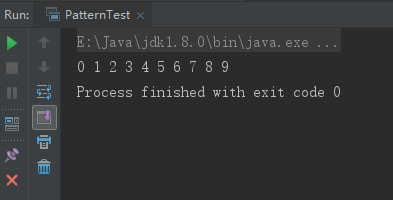9.1可变参数概述与使用

public static void main(String []args){
int arr[]={5,7,4,9,0,1,6,2,8,3};
print(arr);
print(1,2,3);
}
public static void print(int... arr){
for(int i=0;i<arr.length;i++){
System.out.print(arr[i]+" ");
}
System.out.println();
}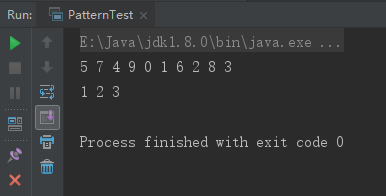9.2Arrays工具类的asList()方法
static List<T> asList(T... a)//返回一个受指定数组支持的固定大小的列表

String str[]={"a","b","c"};
List list= Arrays.asList(str);
System.out.println(list.toString());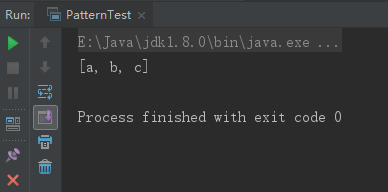ArrayList<ArrayList<Student>> gradeSeven=new ArrayList<>();
ArrayList<Student> classOne=new ArrayList<>();//一班
classOne.add(new Student("一班测试1",20));
classOne.add(new Student("一班测试2",21));
classOne.add(new Student("一班测试3",22));
ArrayList<Student> classTwo=new ArrayList<>();//二班
classTwo.add(new Student("二班测试1",20));
classTwo.add(new Student("二班测试2",21));
//将班级添加到学校中
gradeSeven.add(classOne);
gradeSeven.add(classTwo);
//遍历年级集合，输出年级中的每个人
for(ArrayList<Student> i:gradeSeven){
for(Student j:i){
System.out.println(j);
}
}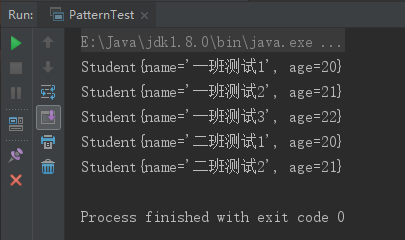## 指针连接两个字符串_极限Redis (3) 动态字符串

Redis是使用C语言开发的，C语言中的字符串大都是通过字符数组实现的，但是使用字符数组有以下不足：字符数组的长度都是固定，容易发生空指针获取字...

## 未定义与 char 类型的输入参数相对应的函数_MATLAB机器人仿真，调用link函数编译错误以及解决方法详解...

MATLAB做机器人仿真，必须要使用的link函数，调用错误以及解决方法MATLAB比较著名的仿真就是simulink，这个仿真使用起来还是比较简单的...